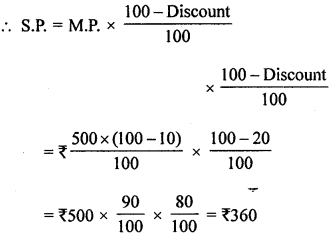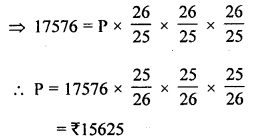## ML Aggarwal Class 8 Solutions for ICSE Maths Model Question Paper 2

Choose the correct answer from the given four options (1—2):
Question 1.
If295x703 is divisible by 11, then value of x is
(a) 5
(b) 6
(c) 7
(d) 8
Solution:
∵ 295×703 is divisible by 11
∴ 3 + 7 + 5 + 2 = 17 and 0 + x + 9 = 9 + x
Their difference = 17 – (9 + x) = 8 – x
8 – x = 0
⇒ x = 8 (d)

Question 2.
If C.P. of an article is ₹500 and S.P. is ₹600, then, his profit % is
(a) 10%
(b) 15%
(c) 20%
(d) 25%
Solution:
C.P. of an article = ₹500
and S.P. = ₹600
Profit = S.P. – C.P. = ₹600 – 500 = ₹100
∴ Profit% = $$\frac{100 \times 100}{500}$$ (Profit % = $$\frac{\text { Profit } \times 100}{\text { C.P. }}$$) = 20% (c)

Question 3.
Find the values of the letters in the following and give reasons for the steps involved:Solution:A + 2 = 9
⇒ A = 9 – 2 = 7
B + 7 = 2 or 12
∴ B = 12 – 7 = 5
∴ A = 7, B = 5

Question 4.
Ramu bought a fan for ₹1080 including 8% VAT. Find the price of fan before VAT was added.
Solution:
C.P. of a fan including 8% VAT = ₹1080
∴ Price of a fan before VATQuestion 5.
Find the amount and compound interest on ₹2000 for 2 years at 5% per annum, interest payable yearly.
Solution:
Principal (P) = ₹2000
Rate (R) = 5% p.a.
Time (n) 2 years
∴ Amount (A) = $$P\left(1+\frac{R}{100}\right)^{n}$$
= ₹2000($$\left(1+\frac{5}{100}\right)^{2}$$
= ₹2200 × $$\frac{21}{20} \times \frac{21}{20}$$ = ₹2205
and C.I. = A – P = ₹2205 – ₹2000 = ₹205

Question 6.
If P = {letters of the word HYDERABAD} and Q= {letters ofthewordALLAHABAD}, find
(i) P ∪ Q
(ii) P ∩ Q
Also verify that
n(P ∪ Q) = n(P) + n(Q) – n(P ∩ Q).
Solution:
P = {letters of the word HYDERABAD} = {H, Y, D, R, E, A, B}
and Q = {letters of the word ALLAHABAD} = {A, L, B, H, D}
(i) P ∪ Q = {H, Y, D, R, L, E, A, R}
(ii) P ∩ Q = {A, H, B, D}

Question 7.
A blanket was marked for ₹500. A shopkeeper allows a discount of 10%. But due to partial damage in the blanket he has to give an extra discount of 20%. Find the S.P. of the blanket.
Solution:
Marked price (M.P.) of a blanket = ₹500
Discount = 10%
Second discount = 20%Question 8.
In a 3 digit number unit’s digit, ten’s digit and hundred’s digit are in the ratio 3 : 5 : 7. If the difference of original number and number obtained by reversing the digits is 396, find the number.
Solution:
In a three digit number,
Ratio in units digit, ten’s digit and hundred’s digit = 3 : 5 : 7
Let unit digit = 3x, then
Tens digit = 5x and
Hundred digit = 7x
Then number = 3x + 5x × 10 + 7x × 100 = 3x + 50x + 700x = 753x
By reversing the order of the digit,  the new number will be 357x
∴ 153x – 357x = 396
⇒ 396x = 396
⇒ x = $$\frac{396}{396}$$ = 1
∴ Number = 753x = 753 × 1 = 753

Question 9.
In an exam atleast 40% marks are required to pass the exam. Rohit uses unfair means to pass the exam but fails by 20 marks. If he scored 220 marks, then find the maximum marks.
Is using unfair means in exam is good? Why should we not use unfair means?
Solution:
Pass percentage = 40%
Rohit scored = 220 marks blit failed by 20 marks
∴ Pass marks = 220 + 20 = 240
∴ 40% of total marks = 240
∴ Maximum marks = $$\frac{240 \times 100}{40}$$ = 600
Using unfair means in an examination is a bad habit and curse on society.
It ruins the life of students. So it should be avoided.

Question 10.
Find the sum invested for $$1 \frac{1}{2}$$ years compounded half-yearly at the rate of 8% p.a. that will amount to ₹ 17576.
Solution:
Amount (A) = ₹17576
Rate (R) = 8% p.a. or 4% half yearly
Period (n) = $$1 \frac{1}{2}$$ years or 3 half yearsHence principal = ₹15625